NEET  >  Revision Notes: Solid State

# Revision Notes: Solid State - Notes | Study NCERT Exemplar & Revision Notes for NEET - NEET

 1 Crore+ students have signed up on EduRev. Have you?

CLASSIFICATION OF SOLIDS
1. Crystalline and  Amorphous solids

Crystalline Solids

• Regular internal arrangement  of particles
• Sharp melting point
• Regarded as true solids
• Undergo regular cut
• Anisotropic in nature

Amorphous solids

• Irregular internal arrangement  of particles
• Melt over a rage of temperature
• Regarded as super cooled liquids or pseudo solids
• Undergo irregular cut.
• Isotropic in nature

2. Based on binding forces
Crystal Classification
(i) Atomic

• Unit Particles - Atoms
• Binding Forces - London dispersion forces
• Properties - Soft, very low melting, poor thermal and electrical conductors
• Examples - Noble gases

(ii) Molecular

• Unit Particles - Polar or non – polar molecules
• Binding Forces - Vander Waal’s forces (London dispersion, dipole – dipole forces hydrogen bonds)
• Properties - Fairly soft, low to moderately high melting points, poor thermal and electrical conductors
• Examples - Dry ice (solid, methane)

(iii) Ionic

• Unit Particles - Positive and negative ions
• Binding Forces - Ionic bonds
• Properties - Hard and brittle, high melting points, high heats of fusion, poor thermal and electrical conductors
• Examples - NaCl, ZnS

(iv) Covalent

• Unit Particles - Atoms that are connected in covalent bond network
• Binding Forces - Covalent bonds
• Properties - Very hard, very high melting points, poor thermal and electrical conductors
• Examples - Diamond, quartz, silicon

(v) Metallic Solids

• Unit Particles - Cations in electron cloud
• Binding Forces - Metallic bonds
• Properties - Soft to very hard, low to very high melting points, excellent thermal and electrical conductors, malleable and ductile
• Examples - All metallic elements, for example, Cu, Fe, Zn

Bragg Equation:
nλ = 2dsinθ,
Where

• d= distance between the planes
• n = order of refraction
• θ= angel of refraction
• λ = wavelength

Crystal Systems:

• Total number of crystal systems: 7
• Total number of Bravais Lattices: 14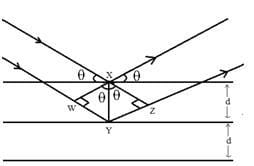CRYSTAL SYSTEMS
(i) Cubic

• Bravais Lattices - Primitive, Face Centered, Body Centered
• Intercepts - a = b = c
• Crystal angle - a = b = g = 90o
• Example - Pb,Hg,Ag,Au Diamond, NaCl, ZnS

(ii) Orthorhombic

• Bravais Lattices - Primitive, Face Centered, Body Centered, End Centered
• Intercepts - a ≠ b ≠ c
• Crystal angle - a = b = g = 90o
• Example - KNO2, K2SO4

(iii) Tetragonal

• Bravais Lattices - Primitive, Body Centered
• Intercepts - a = b ≠ c
• Crystal angle - a = b = g = 90o
• Example - TiO2,SnO2

(iv) Monoclinic

• Bravais Lattices - Primitive, End Centered
• Intercepts - a ≠ b ≠ c
• Crystal angle - a = g = 90o, b≠ 90o
• Example - CaSO4,2H2O

(v) Triclinic

• Bravais Lattices - Primitive
• Intercepts - a ≠ b ≠ c
• Crystal angle - a≠b≠g≠900
• Example - K2Cr2O7, CaSO45H2O

(vi) Hexagonal

• Bravais Lattices - Primitive
• Intercepts - a = b ≠ c
• Crystal angle - a = b = 900, g = 120o
• Example - Mg, SiO2, Zn, Cd

(vii) Rhombohedra

• Bravais Lattices - Primitive
• Intercepts - a = b = c
• Crystal angle - a = g = 90o, b≠ 90o
• Example - As, Sb, Bi, CaCO3

NUMBER OF ATOMS IN UNIT CELLS
Primitive cubic unit cell:

• Number of atoms at corners = 8×1/8 =1
• Number of atoms in faces = 0
• Number of atoms at body-centre: =0
• Total number of atoms = 1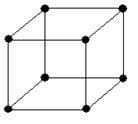Body-centred cubic unit cell:

• Number of atoms at corners = 8×1/8 =1
• Number of atoms in faces = 0
• Number of atoms at body-centre: =1
• Total number of atoms = 2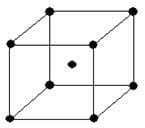Face-centred cubic or cubic-close packed unit cell:

• Number of atoms at corners = 8×1/8 =1
• Number of atoms in faces = 6×1/2 = 3
• Number of atoms at body-centre: = 0
• Total number of atoms = 4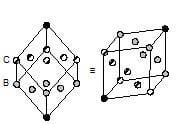PACKING EFFICIENCY
Packing Efficiency = (Volume occupied by all the atoms present in unit cell/Total volume of unit cell)×100

Close structure

(i) hcp and ccp or fcc

• Number of atoms per unit cell ‘z’ - 4
• Relation between edge length ‘a’ and radius of atom ‘r’ - r = a/(2√2)
• Packing Efficiency - 74%

(ii) bcc

• Number of atoms per unit cell ‘z’ - 2
• Relation between edge length ‘a’ and radius of atom ‘r’ - r = (√3/4)a
• Packing Efficiency - 68%

(iii) Simple cubic lattice

• Number of atoms per unit cell ‘z’ - 1
• Relation between edge length ‘a’ and radius of atom ‘r’ - r = a/2
• Packing Efficiency - 52.4%

Density of crystal lattice:
r = (Number of atoms per unit cell × Mass number)/(Volume of unit cell × NA)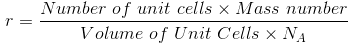or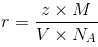Octahedral and Tetrahedral Voids:
Number of octahedral voids = Number of effective atoms present in unit cell
Number of tetrahedral voids = 2×Number of effective atoms present in unit cell
So, Number of tetrahedral voids = 2× Number of octahedral voids.

Coordination numbers

(i) 2

• Geometry - Linear
• Radius ratio (x) - x < 0.155
• Example - BeF2

(ii) 3

• Geometry - Planar Triangle
• Radius ratio (x) - 0.155 ≤ x < 0.225
• Example - AlCl3

(iii) 4

• Geometry - Tetrahedron
• Radius ratio (x) - 0.225 ≤ x < 0.414
• Example - ZnS

(iv) 5

• Geometry - Square planar
• Radius ratio (x) - 0.414 ≤ x < 0.732
• Example - PtCl42-

(v) 6

• Geometry - Octahedron
• Radius ratio (x) - 0.414 ≤ x < 0.732
• Example - NaCl

(vi) 8

• Geometry - Body centered cubic
• Radius ratio (x) - 0.732 ≤ x < 0.999
• Example - CsCl

CLASSIFICATION OF IONIC STRUCTURES
Structures

(i) Rock Salt Structure

• Descriptions - Anion(Cl-) forms fcc units and cation(Na+) occupy octahedral voids. Z=4 Coordination number =6
• Examples - NaCl, KCl, LiCl, RbCl

(ii) Zinc Blende Structure

• Descriptions - Anion (S2-) forms fcc units and cation (Zn2+) occupy alternate tetrahedral voids Z=4 Coordination number =4
• Examples - ZnS , BeO

(iii) Fluorite Structures

• Descriptions - Cation (Ca2+) forms fcc units and anions (F-) occupy tetrahedral voids Z= 4 Coordination number of anion = 4 Coordination number of cation = 8
• Examples - CaF2, UO2, and ThO2

(iv) Anti- Fluorite Structures

• Descriptions - Oxide ions are face centered and metal ions occupy all the tetrahedral voids.
• Examples - Na2O, K2O and Rb2O.

(v) Cesium Halide Structure

• Descriptions - Halide ions are primitive cubic while the metal ion occupies the center of the unit cell. Z=2 Coordination number of = 8
• Examples - All Halides of Cesium.

(vi) Pervoskite Structure

• Descriptions - One of the cation is bivalent and the other is tetravalent. The bivalent ions are present in primitive cubic lattice with oxide ions on the centers of all the six square faces. The tetravalent cation is in the center of the unit cell occupying octahedral void.
• Examples - CaTiO3, BaTiO3

(vii) Spinel and Inverse Spinel Structure

• Descriptions - Spinel :M2+M23+O4, where M2+ is present in one-eighth of tetrahedral voids in a FCC lattice of oxide ions and M3+ ions are present in half of the octahedral voids. M2+ is usually Mg, Fe, Co, Ni, Zn and Mn;  M3+ is generally Al, Fe, Mn, Cr and Rh.
• Examples - MgAl2O4 , ZnAl2O4, Fe3O4,FeCr2O4 etc.

DEFECTS IN CRYSTAL
Stoichiometric Defects
1. Schottky Defects

•  Some of the lattice points in a crystal are unoccupied.
• Appears in ionic compounds in which anions and cations are of nearly same size.
• Decreases the density of lattice
• Examples: NaCl and KCl

2.  Frenkel Defects

• Ion dislocate from its position and occupies an interstitial position between the lattice points
• Appears in crystals in which the negative ions are much larger than the positive ion.
• Does not affect density of the crystal.
• Examples: AgBr, ZnS

NON-STOICHIOMETRIC DEFECTS
1. Metal Excess defect:
Metal excess defect occurs due to

• anionic vacancies or
• presence of extra cation.
• F-Centres: hole produced due to absence of anion which is occupied by an electron.

2. Metal deficiency defect:
Metal deficiency defect occurs

• due to variable valency of metals
• when one of the positive ions is missing from its lattice site and the extra negative charge is balanced by some nearby metal ion acquiring two charges instead of one
The document Revision Notes: Solid State - Notes | Study NCERT Exemplar & Revision Notes for NEET - NEET is a part of the NEET Course NCERT Exemplar & Revision Notes for NEET.
All you need of NEET at this link: NEET

## NCERT Exemplar & Revision Notes for NEET

291 docs
 Use Code STAYHOME200 and get INR 200 additional OFF

## NCERT Exemplar & Revision Notes for NEET

291 docs

### How to Prepare for NEET

Read our guide to prepare for NEET which is created by Toppers & the best Teachers

Track your progress, build streaks, highlight & save important lessons and more!

,

,

,

,

,

,

,

,

,

,

,

,

,

,

,

,

,

,

,

,

,

;